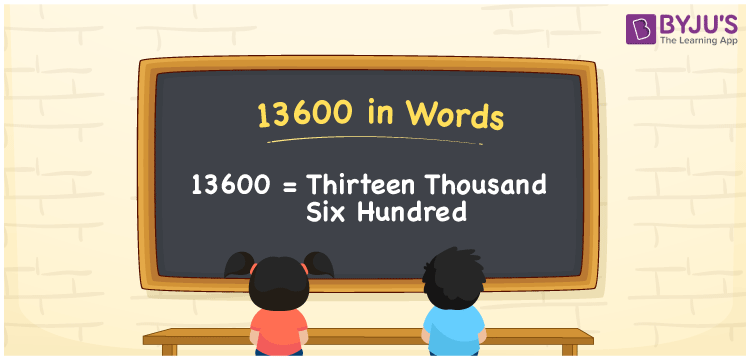# 13600 in Words

Thirteen thousand six hundred is the word for the number 13600. Using the place value of a number, the number 13600 can be easily transformed into words. As a result, you’ll need to create the number names using a place value table. For example, if you paid Rs. 13600 for a table, you could write, “I paid Rs. Thirteen thousand six hundred for a table”. In this topic, you will learn how to spell 13600 in English as well as how to describe the number in words.

 13600 in Words: Thirteen Thousand Six Hundred. Thirteen Thousand Six Hundred in Numerical Form: 13600.

## 13600 in English Words## How to Write 13600 in Words?

The following table represents the place value for the number 13600.

 Ten-thousands Thousands Hundreds Tens Ones 1 3 6 0 0

The number 13600 in its expanded form as follows:

= 1× Ten thousand + 3 × Thousand + 6 × Hundred + 0 × Ten + 0 × One

= 1 × 10000 + 3 × 1000 + 6 × 100

= 10000 + 3000 + 600

= 13600

= Thirteen thousand six hundred

Hence, 13600 in words is Thirteen thousand six hundred.

13600 in words – Thirteen thousand six hundred

Is 13600 an odd number? – No

Is 13600 an even number? – Yes

Is 13600 a perfect square number? – No

Is 13600 a perfect cube number? – No

Is 13600 a prime number? – No

Is 13600 a composite number? – Yes

## Frequently Asked Questions on 13600 in Words

Q1

### Write 13600 in words.

13600 in words can be written as thirteen thousand six hundred.

Q2

### Simplify 14000 – 400, and express in words.

Simplifying 14000 – 400, we get 13600. Hence, 13600 in words is written as thirteen thousand six hundred.

Q3

### How to write thirteen thousand six hundred in numbers?

Thirteen thousand six hundred in numbers is 13600

Test your Knowledge on 13600 in Words